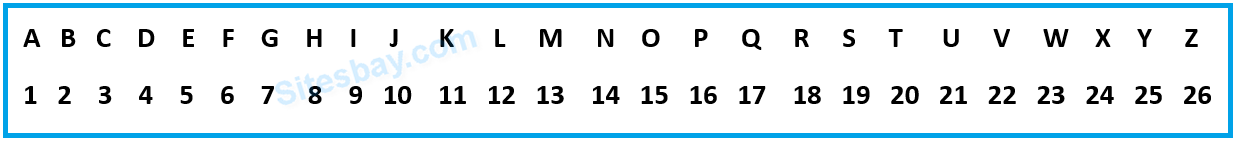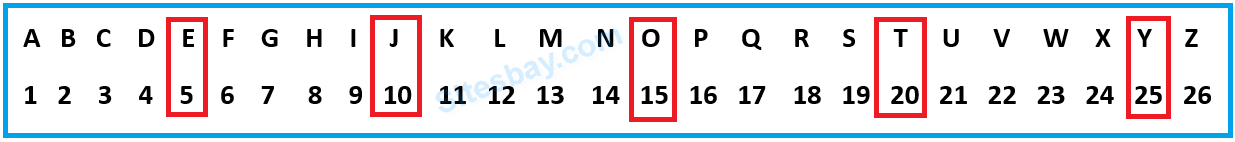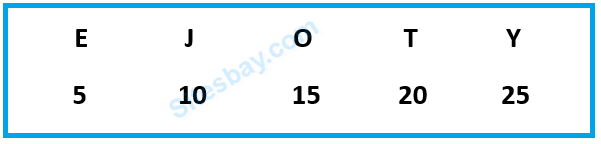# Alphabet Series Tricks

## Alphabet Series Reasoning Tricks

Before beginning the Alphabet Series Let's give me answer of one question find the missing term in the 1, 4, 6, 8, ____ series. You can easily know next number is 10.

Similar thing can be asked in Alphabet Series like; A, D, F, H, ____. Here answer would be 'J' because you understood it quickly that like the number series you need to skip two letter and reach the next letter.

To resolve this type of question remember all the alphabets and their place number. You can even note down during your exam.Here you should remember two thingsAlso Remember these intervals,It would be helpful in solving alphabet series problems.### What is the value of V?

So here in this case you can tell immediately that it is 22 because you know that immediately after U is V and its value is 22.

### What is the missing letter in the series? U O I ___ A.

Solution: If you observe it attentively, then we can see that they are the vowels written in the REVERSE order. U O I E A and answer is E

## Solution

```Enter First number: 6
To do this we need to see their numeral values

A D  =  1 and 1+3 = 1 4

G J  =4+3  is 7 and 7+3 is 10 = 7 10

MP = 10+3 is 13 and 13+3 is 16 = 13 16

So the next would be 16+3 is 19 which means S and 19+3 is 22 which means V = SV

The missing term is SV.
```